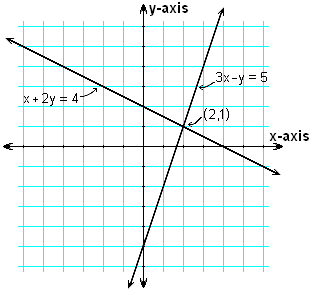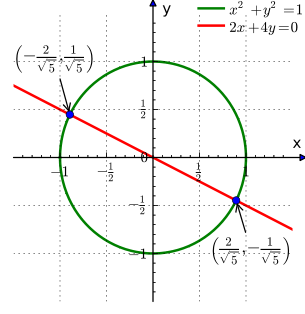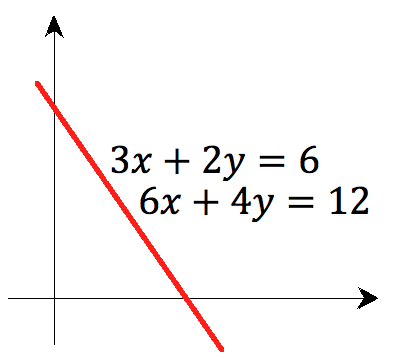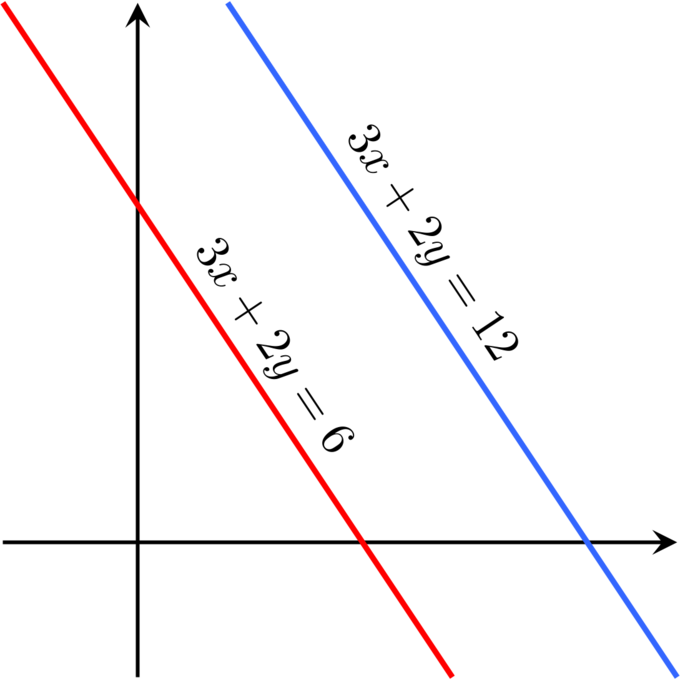## Introduction to Systems of Equations

A system of equations consists of two or more equations with two or more variables, where any solution must satisfy all of the equations in the system at the same time.

### Learning Objectives

Explain what systems of equations can represent

### Key Takeaways

#### Key Points

• A system of linear equations consists of two or more linear equations made up of two or more variables, such that all equations in the system are considered simultaneously.
• To find the unique solution to a system of linear equations, we must find a numerical value for each variable in the system that will satisfy all equations in the system at the same time.
• In order for a linear system to have a unique solution, there must be at least as many equations as there are variables.
• The solution to a system of linear equations in two variables is any ordered pair $(x, y)$ that satisfies each equation independently. Graphically, solutions are points at which the lines intersect.

#### Key Terms

• system of linear equations: A set of two or more equations made up of two or more variables that are considered simultaneously.
• dependent system: A system of linear equations in which the two equations represent the
same line; there are an infinite number of solutions to a dependent system.
• inconsistent system: A system of linear equations with no common solution because they
represent parallel lines, which have no point or line in common.
• independent system: A system of linear equations with exactly one solution pair $(x, y)$.

A system of linear equations consists of two or more linear equations made up of two or more variables, such that all equations in the system are considered simultaneously. To find the unique solution to a system of linear equations, we must find a numerical value for each variable in the system that will satisfy all of the system’s equations at the same time. Some linear systems may not have a solution, while others may have an infinite number of solutions. In order for a linear system to have a unique solution, there must be at least as many equations as there are variables. Even so, this does not guarantee a unique solution.

In this section, we will focus primarily on systems of linear equations which consist of two equations that contain two different variables. For example, consider the following system of linear equations in two variables:

$2x + y = 15 \\ 3x - y = 5$

The solution to a system of linear equations in two variables is any ordered pair that satisfies each equation independently. In this example, the ordered pair (4, 7) is the solution to the system of linear equations. We can verify the solution by substituting the values into each equation to see if the ordered pair satisfies both equations.

$2(4) + 7 = 15 \\ 3(4) - 7 = 5$

Both of these statements are true, so $(4, 7)$ is indeed a solution to the system of equations.

Note that a system of linear equations may contain more than two equations, and more than two variables. For example,

$3x + 2y - z = 12 \\ x - 2y + 4z = -2 \\ -x + 12y -z = 0$

is a system of three equations in the three variables $x, y, z$. A solution to the system above is given by

$x = 1 \\ y = -2 \\ z = - 2$

since it makes all three equations valid.

### Types of Linear Systems and Their Solutions

In general, a linear system may behave in any one of three possible ways:

1. The system has a single unique solution.
2. The system has no solution.
3. The system has infinitely many solutions.

Each of these possibilities represents a certain type of system of linear equations in two variables. Each of these can be displayed graphically, as below. Note that a solution to a system of linear equations is any point at which the lines intersect.Systems of Linear Equations: Graphical representations of the three types of systems.

An independent system has exactly one solution pair $(x, y)$. The point where the two lines intersect is the only solution.

An inconsistent system has no solution. Notice that the two lines are parallel and will never intersect.

A dependent system has infinitely many solutions. The lines are exactly the same, so every coordinate pair on the line is a solution to both equations.

## Solving Systems Graphically

A simple way to solve a system of equations is to look for the intersecting point or points of the equations. This is the graphical method.

### Learning Objectives

Solve a system of equations in two variables graphically

### Key Takeaways

#### Key Points

• To solve a system of equations graphically, graph the equations and identify the points of intersection as the solutions. There can be more than one solution to a system of equations.
• A system of linear equations will have one point of intersection, or one solution.
• To graph a system of equations that are written in standard form, you must rewrite the equations in slope -intercept form.

#### Key Terms

• system of equations: A set of equations with multiple variables which can be solved using a specific set of values.
• The graphical method: A way of visually finding a set of values that solves a system of equations.

A system of equations (also known as simultaneous equations) is a set of equations with multiple variables, solved when the values of all variables simultaneously satisfy all of the equations. The most common ways to solve a system of equations are:

• The graphical method
• The substitution method
• The elimination method

Here, we will address the graphical method.

### Solving Systems Graphically

Some systems have only one set of correct answers, while others have multiple sets that will satisfy all equations. Shown graphically, a set of equations solved with only one set of answers will have only have one point of intersection, as shown below. This point is considered to be the solution of the system of equations. In a set of linear equations (such as in the image below), there is only one solution.System of linear equations with two variables: This graph shows a system of equations with two variables and only one set of answers that satisfies both equations.

A system with two sets of answers that will satisfy both equations has two points of intersection (thus, two solutions of the system), as shown in the image below.System of equations with multiple answers: This is an example of a system of equations shown graphically that has two sets of answers that will satisfy both equations in the system.

### Converting to Slope-Intercept Form

Before successfully solving a system graphically, one must understand how to graph equations written in standard form, or $Ax+By=C$. You can always use a graphing calculator to represent the equations graphically, but it is useful to know how to represent such equations formulaically on your own.

To do this, you need to convert the equations to slope-intercept form, or $y=mx+b$, where m = slope and b = y-intercept.

The best way to convert an equation to slope-intercept form is to first isolate the y variable and then divide the right side by B, as shown below.

\begin{align} \displaystyle Ax+By&=C \\By&=-Ax+C \\y&=\frac{-Ax+C}{B} \\y&=-\frac{A}{B}x+\frac{C}{B} \end{align}

Now $\displaystyle -\frac{A}{B}$ is the slope m, and $\displaystyle \frac{C}{B}$ is the y-intercept b.

### Identifying Solutions on a Graph

Once you have converted the equations into slope-intercept form, you can graph the equations. To determine the solutions of the set of equations, identify the points of intersection between the graphed equations. The ordered pair that represents the intersection(s) represents the solution(s) to the system of equations.

## The Substitution Method

The substitution method is a way of solving a system of equations by expressing the equations in terms of only one variable.

### Learning Objectives

Solve systems of equations in two variables using substitution

### Key Takeaways

#### Key Points

• A system of equations is a set of equations that can be solved using a particular set of values.
• The substitution method works by expressing one of the variables in terms of another, then substituting it back into the original equation and simplifying it.
• It is very important to check your work once you have found a set of values for the variables. Do this by substituting the values you found back into the original equations.
• The solution to the system of equations can be written as an ordered pair (x,y).

#### Key Terms

• substitution method: Method of solving a system of equations by putting the equation in terms of only one variable
• system of equations: A set of equations with multiple variables which can be solved using a specific set of values.

The substitution method for solving systems of equations is a way to simplify the system of equations by expressing one variable in terms of another, thus removing one variable from an equation. When the resulting simplified equation has only one variable to work with, the equation becomes solvable.

The substitution method consists of the following steps:

1. In the first equation, solve for one of the variables in terms of the others.
2. Substitute this expression into the remaining equations.
3. Continue until you have reduced the system to a single linear equation.
4. Solve this equation, and then back-substitute until the solution is found.

### Solving with the Substitution Method

Let’s practice this by solving the following system of equations:

$x-y=-1$

$x+2y=-4$

We begin by solving the first equation so we can express x in terms of y.

\begin{align} \displaystyle x-y&=-1 \\x&=y-1 \end{align}

Next, we will substitute our new definition of x into the second equation:

\displaystyle \begin{align} x+2y&=-4 \\(y-1)+2y&=-4 \end{align}

Note that now this equation only has one variable (y). We can then simplify this equation and solve for y:

\displaystyle \begin{align} (y-1)+2y&=-4 \\3y-1&=-4 \\3y&=-3 \\y&=-1 \end{align}

Now that we know the value of y, we can use it to find the value of the other variable, x. To do this, substitute the value of y into the first equation and solve for x.

\displaystyle \begin{align} x-y&=-1 \\x-(-1)&=-1 \\x+1&=-1 \\x&=-1-1 \\x&=-2 \end{align}

Thus, the solution to the system is: $(-2, -1)$, which is the point where the two functions graphically intersect. Check the solution by substituting the values into one of the equations.

\displaystyle \begin{align} x-y&=-1 \\(-2)-(-1)&=-1 \\-2+1&=-1 \\-1&=-1 \end{align}

## The Elimination Method

The elimination method is used to eliminate a variable in order to more simply solve for the remaining variable(s) in a system of equations.

### Learning Objectives

Solve systems of equations in two variables using elimination

### Key Takeaways

#### Key Points

• The steps of the elimination method are: (1) set the equations up so the variables line up, (2) modify one equation so both equations share a consistent variable that can be eliminated, (3) add the equations together to eliminate the variable, (4) solve, and (5) back-substitute to solve for the other variable.
• Always check the answer. This is done by plugging both values into one or both of the original equations.

#### Key Terms

• elimination method: Process of solving a system of equations by eliminating one variable in order to more simply solve for the remaining variable.
• system of equations: A set of equations with multiple variables which can be solved using a specific set of values.

The elimination method for solving systems of equations, also known as elimination by addition, is a way to eliminate one of the variables in the system in order to more simply evaluate the remaining variable. Once the values for the remaining variables have been found successfully, they are substituted into the original equation in order to find the correct value for the other variable.

The elimination method follows these steps:

1. Rewrite the equations so the variables line up.
2. Modify one equation so both equations have a variable that will cancel itself out when the equations are added together.
3. Add the equations and eliminate the variable.
4. Solve for the remaining variable.
5. Back-substitute and solve for the other variable.

### Solving with the Elimination Method

The elimination method can be demonstrated by using a simple example:

$\displaystyle 4x+y=8 \\ 2y+x=9$

First, line up the variables so that the equations can be easily added together in a later step:

\displaystyle \begin{align} 4x+y&=8 \\x+2y&=9 \end{align}

Next, look to see if any of the variables are already set up in such a way that adding them together will cancel them out of the system. If not, multiply one equation by a number that allow the variables to cancel out. In this example, the variable y can be eliminated if we multiply the top equation by $-2$ and then add the equations together.

Multiplication step:

\displaystyle \begin{align} -2(4x+y&=8) \\x+2y&=9 \end{align}

Result:

\displaystyle \begin{align} -8x-2y&=-16 \\x+2y&=9 \end{align}

Now add the equations to eliminate the variable y.

\displaystyle \begin{align} -8x+x-2y+2y&=-16+9 \\-7x&=-7 \end{align}

Finally, solve for the variable x.

\displaystyle \begin{align} -7x&=-7 \\x&=\frac{-7}{-7} \\x&=1 \end{align}

Then go back to one of the original equations and substitute the value we found for x. It is easiest to pick the simplest equation, but either equation will work.

\displaystyle \begin{align} 4x+y&=8 \\4(1)+y&=8 \\4+y&=8 \\y&=4 \end{align}

Therefore, the solution of the equation is (1,4). It is always important to check the answer by substituting both of these values in for their respective variables into one of the equations.

\displaystyle \begin{align} 4x+y&=8 \\4(1)+4&=8 \\4+4&=8 \\8&=8 \end{align}

## Inconsistent and Dependent Systems in Two Variables

For linear equations in two variables, inconsistent systems have no solution, while dependent systems have infinitely many solutions.

### Learning Objectives

Explain when systems of equations in two variables are inconsistent or dependent both graphically and algebraically.

### Key Takeaways

#### Key Points

• Graphically, the equations in a dependent system represent the same line. The equations in an inconsistent system represent parallel lines that never intersect.
• We can use methods for solving systems of equations to identify dependent and inconsistent systems: Dependent systems have an infinite number of solutions. Applying methods of solving systems of equations will result in a true identity, such as $0 = 0$. Inconsistent systems have no solutions. Applying methods of solving systems of equations will result in a contradiction, such as the statement $0 = 1$.

#### Key Terms

• inconsistent system: A system of linear equations with no common solution because they
represent parallel lines, which have no point or line in common.
• independent system: A system of linear equations with exactly one solution pair.
• dependent system: A system of linear equations in which the two equations represent the
same line; there are an infinite number of solutions to a dependent system.

Recall that a linear system may behave in any one of three possible ways:

1. The system has a single unique solution.
2. The system has no solution.
3. The system has infinitely many solutions.

Also recall that each of these possibilities corresponds to a type of system of linear equations in two variables. An independent system of equations has exactly one solution $(x,y)$. An inconsistent system has no solution, and a dependent system has an infinite number of solutions.

The previous modules have discussed how to find the solution for an independent system of equations. We will now focus on identifying dependent and inconsistent systems of linear equations.

### Dependent Systems

The equations of a linear system are independent if none of the equations can be derived algebraically from the others. When the equations are independent, each equation contains new information about the variables, and removing any of the equations increases the size of the solution set. Systems that are not independent are by definition dependent. Equations in a dependent system can be derived from one another; they describe the same line. They do not add new information about the variables, and the loss of an equation from a dependent system does not change the size of the solution set.

We can apply the substitution or elimination methods for solving systems of equations to identify dependent systems. Dependent systems have an infinite number of solutions because all of the points on one line are also on the other line. After using substitution or addition, the resulting equation will be an identity, such as $0 = 0$.

For example, consider the two equations

$3x+2y = 6 \\ 6x+4y = 12$

We can apply the elimination method to evaluate these. If we were to multiply the first equation by a factor of $-2$, we would have:

\displaystyle \begin{align} -2(3x+2y&=6) \\-6x-4y&=-12 \end{align}

Adding this to the second equation would yield $0=0$. Thus, the two lines are dependent. Also note that they are the same equation scaled by a factor of two; in other words, the second equation can be derived from the first.

When graphed, the two equations produce identical lines, as demonstrated below.Dependent system: The equations $3x + 2y = 6$ and $6x + 4y = 12$ are dependent, and when graphed produce the same line.

Note that there are an infinite number of solutions to a dependent system, and these solutions fall on the shared line.

### Inconsistent Systems

A linear system is consistent if it has a solution, and inconsistent otherwise. Recall that the graphical representation of an inconsistent system consists of parallel lines that have the same slope but different $y$-intercepts. They will never intersect.

We can also apply methods for solving systems of equations to identify inconsistent systems. When the system is inconsistent, it is possible to derive a contradiction from the equations, such as the statement $0 = 1$.

Consider the following two equations:

$3x+2y = 6 \\ 3x+2y = 12$

We can apply the elimination method to attempt to solve this system. Subtracting the first equation from the second one, both variables are eliminated and we get $0 = 6$. This is a contradiction, and we are able to identify that this is an inconsistent system. The graphs of these equations on the $xy$-plane are a pair of parallel lines.Inconsistent system: The equations $3x + 2y = 6$ and $3x + 2y = 12$ are inconsistent.

In general, inconsistencies occur if the left-hand sides of the equations in a system are linearly dependent, and the constant terms do not satisfy the dependence relation. A system of equations whose left-hand sides are linearly independent is always consistent.

## Applications of Systems of Equations

Systems of equations can be used to solve many real-life problems in which multiple constraints are used on the same variables.

### Learning Objectives

Apply systems of equations in two variables to real world examples

### Key Takeaways

#### Key Points

• If you have a problem that includes multiple variables, you can solve it by creating a system of equations.
• Once variables are defined, determine the relationships between them and write them as equations.

#### Key Terms

• system of equations: A set of equations with multiple variables which can be solved using a specific set of values.

#### Systems of Equations in the Real World

A system of equations, also known as simultaneous equations, is a set of equations that have multiple variables. The answer to a system of equations is a set of values that satisfies all equations in the system, and there can be many such answers for any given system. Answers are generally written in the form of an ordered pair: $\left( x,y \right)$. Approaches to solving a system of equations include substitution and elimination as well as graphical techniques.

There are several practical applications of systems of equations. These are shown in detail below.

### Planning an Event

A system of equations can be used to solve a planning problem where there are multiple constraints to be taken into account:

Emily is hosting a major after-school party. The principal has imposed two restrictions. First, the total number of people attending (teachers and students combined) must be $56$. Second, there must be one teacher for every seven students. So, how many students and how many teachers are invited to the party?

First, we need to identify and name our variables. In this case, our variables are teachers and students. The number of teachers will be $T$, and the number of students will be $S$.

Now we need to set up our equations. There is a constraint limiting the total number of people in attendance to $56$, so:

$T+S=56$

For every seven students, there must be one teacher, so:

$\frac{S}{7}=T$

Now we have a system of equations that can be solved by substitution, elimination, or graphically. The solution to the system is $S=49$ and $T=7$.

### Finding Unknown Quantities

This next example illustrates how systems of equations are used to find quantities.

A group of $75$ students and teachers are in a field, picking sweet potatoes for the needy. Kasey picks three times as many sweet potatoes as Davis—and then, on the way back to the car, she picks up five more! Looking at her newly increased pile, Davis remarks, “Wow, you’ve got $29$ more potatoes than I do!” How many sweet potatoes did Kasey and Davis each pick?

To solve, we first define our variables. The number of sweet potatoes that Kasey picks is $K$, and the number of sweet potatoes that Davis picks is $D$.

Now we can write equations based on the situation:

$K-5 = 3D$

$D+29 = K$

From here, substitution, elimination, or graphing will reveal that $K=41$ and $D=12$.

It is important that you always check your answers. A good way to check solutions to a system of equations is to look at the functions graphically and then see where the graphs intersect. Or, you can substitute your answers into every equation and check that they result in accurate solutions.

### Other Applications

There are a multitude of other applications for systems of equations, such as figuring out which landscaper provides the best deal, how much different cell phone providers charge per minute, or comparing nutritional information in recipes.